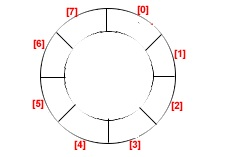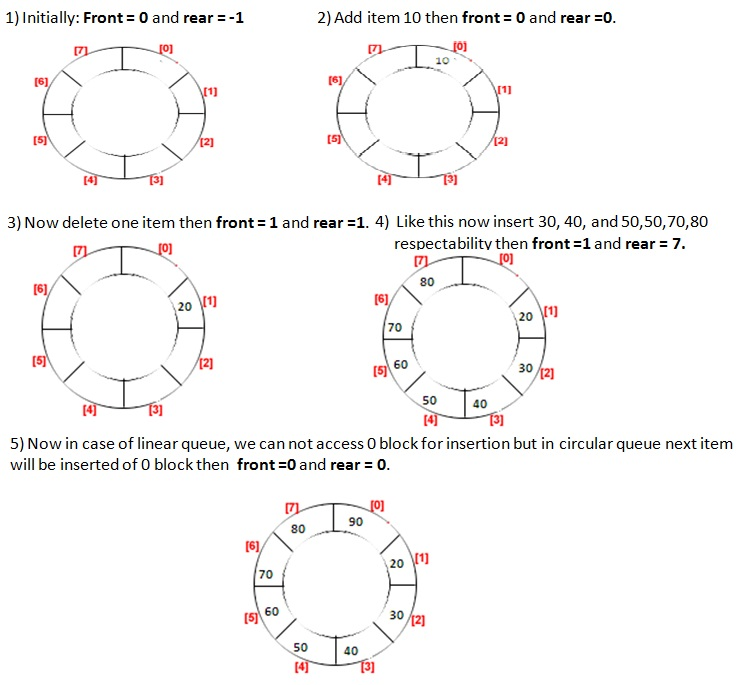# Circular Queue Tutorial

## What is Circular Queue?

The queue is considered as a circular queue when the positions 0 and MAX-1 are adjacent. Any position before front is also after rear.

A circular queue looks likeNote:
Note that the container of items is an array. Array is stored in main memory. Main memory is linear. So this circularity is only logical. There can not be physical circularity in main memory.

Consider the example with Circular Queue implementationSee the logical circularity of the queue. Addition causes the increment in REAR. It means that when REAR reaches MAX-1 position then Increment in REAR causes REAR to reach at first position that is 0.

```    if( rear  == MAX -1 )
rear  = 0;
else
rear = rear + 1;
```

The short-hand equivalent representation may be

```	rear = ( rear + 1) % MAX;
```

As we know that, Deletion causes the increment in FRONT. It means that when FRONT reaches the MAX-1 position, then increment in FRONT, causes FRONT to reach at first position that is 0.

```    if( front  == MAX -1 )
front  = 0;
else
front = front + 1;
```

The short-hand equivalent representation may be

```	front = ( front + 1) % MAX;
```

### Drawback of Circular Queue

The drawback of circular queue is , difficult to distinguished the full and empty cases. It is also known as boundary case problem.

In circular queue it is necessary that:

• Before insertion, fullness of Queue must be checked (for overflow).
• Before deletion, emptiness of Queue must be checked (for underflow).

Condition to check FULL Circular Queue

```    if( ( front == MAX-1) || ( front ==0 && rear == MAX -1 ) )
```
Condition to check EMPTY Circular Queue
```    if( ( front == MAX-1) || ( front ==0 && rear == MAX -1 ) )
```

### Solution of the drawback of circular Queue

Use count variable to hold the current position ( in case of insertion or deletion).

### Operation of Circular Queue using count

1. Initialize Operation.
2. Is_Full check.
3. Is_Empty check.
5. Deletion operation.

#### Algorithms

1. INIT(QUEUE,FRONT,REAR,COUNT)
2. INSERT-ITEM(QUEUE, FRONT, REAR, MAX, COUNT, ITEM)
3. REMOVE-ITEM(QUEUE, FRONT, REAR, COUNT, ITEM)
4. FULL-CHECK(QUEUE,FRONT,REAR,MAX,COUNT,FULL)
5. EMPTY-CHECK(QUEUE,FRONT,REAR,MAX,COUNT,EMPTY)
```    INIT(QUEUE,FORNT,REAR,COUNT)
This algorithm is used to initialize circular queue.
1.	FRONT := 1;
2.	REAR  := 0;
3.	COUNT :=  0;
4.	Return;
```
```INSERT-ITEM( QUEUE, FRONT, REAR, MAX, COUNT, ITEM)
This algorithm is used to insert or add item
into circular queue.
1.	If ( COUNT = MAX ) then
a.	Display  “Queue overflow”;
b.	Return;
2.	Otherwise
a.	If ( REAR = MAX ) then
i.	REAR := 1;
b.	Otherwise
i.	REAR := REAR + 1;
c.	QUEUE(REAR) := ITEM;
d.	COUNT := COUNT + 1;
3.	Return;
```
```REMOVE-ITEM( QUEUE, FRONT, REAR, COUNT, ITEM)
This algorithm is used to remove or delete item
from circular queue.
1.	If ( COUNT = 0 ) then
a.	Display “Queue underflow”;
b.	Return;
2.	Otherwise
a.	ITEM := QUEUE(FRONT)l
b.	If ( FRONT =MAX ) then
i.	FRONT := 1;
c.	Otherwise
i.	FRONT := FRONT + 1;
d.	COUNT := COUNT + 1;
3.	Return;
```
```EMPTY-CHECK(QUEUE,FRONT,REAR,MAX,COUNT,EMPTY)
This is used to check queue is empty or not.
1.	If( COUNT = 0 ) then
a.	EMPTY := true;
2.	Otherwise
a.	EMPTY := false;
3.	Return ;
```
```FULL-CHECK(QUEUE,FRONT,REAR,MAX,COUNT,FULL)
This algorithm is used to check queue is full or not.
1.	If ( COUNT = MAX ) then
a.	FULL := true;
2.	Otherwise
a.	FULL := false;
3.	Return ;
```

#### Declaration of Circular Queue

```#define MAX 5
/*Declaration of circular queue.*/
typedef struct
{
int front	;
int rear	;
int count	;
int ele[MAX]	;
}CirQueue;
```
```#define MAX 5

//Declaration of Circular Queue
class CirQueue
{
private:
int front	;
int rear	;
int count	;
int ele[MAX]	;

public:
CirQueue();
int  isFull();
int  isEmpty();
void insertQueue(int item);
int  deleteQueue(int *item);
};
```

#### Circular Queue Implementation for all above queue operations

```#include <stdio.h>

#define MAX 5

/*Declaration of circular queue.*/
typedef struct
{
int front	;
int rear	;
int count	;
int ele[MAX]	;
}CirQueue;

/*Initailization of circular queue.*/
void initCirQueue(CirQueue * q)
{
q->front =  0;
q->rear  = -1;
q->count =  0;
}

/*Check Queue is full or not*/
int isFull(CirQueue * q)
{
int full=0;

if(q->count == MAX)
full = 1;

return full;
}

/*Check Queue is empty or not*/
int isEmpty(CirQueue * q)
{
int empty=0;

if(q->count == 0)
empty = 1;

return empty;
}

/*To insert item into circular queue.*/
void insertCirQueue(CirQueue * q, int item)
{
if( isFull(q) )
{
printf("\nQueue Overflow");
return;
}

q->rear = (q->rear+1)%MAX;
q->ele[q->rear] = item;

q->count++;

printf("\nInserted item : %d",item);
}

/*To delete item from queue.*/
int deleteCirQueue(CirQueue * q, int *item)
{
if( isEmpty(q) )
{
printf("\nQueue Underflow");
return -1;
}

*item    = q->ele[q->front];

q->front = (q->front+1)%MAX;

q->count--;

return 0;
}

int main()
{
int item=0;
CirQueue q;

initCirQueue(&q);

insertCirQueue(&q, 10);
insertCirQueue(&q, 20);
insertCirQueue(&q, 30);
insertCirQueue(&q, 40);
insertCirQueue(&q, 50);
insertCirQueue(&q, 60);

if ( deleteCirQueue( &q, &item ) == 0 )
printf("\nDeleted item is : %d",item);

if ( deleteCirQueue( &q, &item ) == 0 )
printf("\nDeleted item is : %d",item);

if ( deleteCirQueue( &q, &item ) == 0 )
printf("\nDeleted item is : %d",item);

if ( deleteCirQueue( &q, &item ) == 0 )
printf("\nDeleted item is : %d",item);

if ( deleteCirQueue( &q, &item ) == 0 )
printf("\nDeleted item is : %d",item);

insertCirQueue(&q, 60);

if ( deleteCirQueue( &q, &item ) == 0 )
printf("\nDeleted item is : %d",item);

if ( deleteCirQueue( &q, &item ) == 0 )
printf("\nDeleted item is : %d",item);

printf("\n");
return 0;
}
```
```    Inserted item : 10
Inserted item : 20
Inserted item : 30
Inserted item : 40
Inserted item : 50
Queue Overflow
Deleted item is : 10
Deleted item is : 20
Deleted item is : 30
Deleted item is : 40
Deleted item is : 50
Inserted item : 60
Deleted item is : 60
Queue Underflow
```
```#include <iostream>

using namespace std;
#define MAX 5

//Declaration of Circular Queue
class CirQueue
{
private:
int front	;
int rear	;
int count	;
int ele[MAX]	;

public:
CirQueue();
int  isFull();
int  isEmpty();
void insertQueue(int item);
int  deleteQueue(int *item);
};

//Initalize Circular Queue
CirQueue:: CirQueue()
{
front =  0;
rear  = -1;
count =  0;
}

//To check queue is full or not
int CirQueue:: isFull()
{
int full=0;

if( count == MAX )
full = 1;

return full;
}

//To check queue is empty or not
int CirQueue:: isEmpty()
{
int empty=0;

if( count == 0 )
empty = 1;

return empty;
}

//Insert item into circular queue
void CirQueue:: insertQueue(int item)
{
if( isFull() )
{
cout<<"\nQueue Overflow";
return;
}

rear= (rear+1) % MAX;

ele[rear] = item;
count++;

cout<<"\nInserted item : "<< item;
}

//Delete item from circular queue
int CirQueue:: deleteQueue(int *item)
{
if( isEmpty() )
{
cout<<"\nQueue Underflow";
return -1;
}
*item = ele[front];
front = (front+1) % MAX;
count--;
return 0;
}

int main()
{
int  item;
CirQueue q = CirQueue();

q.insertQueue(10);
q.insertQueue(20);
q.insertQueue(30);
q.insertQueue(40);
q.insertQueue(50);
q.insertQueue(60);

if( q.deleteQueue(&item) == 0 )
{
cout<<"\nDeleted item:"<< item<< endl;
}

if( q.deleteQueue(&item) == 0 )
{
cout<<"\nDeleted item:"<< item<< endl;
}

if( q.deleteQueue(&item) == 0 )
{
cout<<"\nDeleted item:"<< item << endl;
}

if( q.deleteQueue(&item) == 0 )
{
cout<<"\nDeleted item:"<< item<< endl;
}

if( q.deleteQueue(&item) == 0 )
{
cout<<"\nDeleted item:"<< item<< endl;
}

q.insertQueue(60);
if( q.deleteQueue(&item) == 0 )
{
cout<<"\nDeleted item:"<< item<< endl;
}

if( q.deleteQueue(&item) == 0 )
{
cout<<"\nDeleted item:"<< item<< endl;
}

cout<<"\n";
return 0;
}

```
```    Inserted item : 10
Inserted item : 20
Inserted item : 30
Inserted item : 40
Inserted item : 50
Queue Overflow
Deleted item:10

Deleted item:20

Deleted item:30

Deleted item:40

Deleted item:50

Inserted item : 60
Deleted item:60

Queue Underflow
```